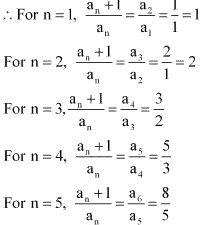# Class 11 Maths NCERT Solutions for Chapter 9 Sequences and Series Exercise 9.1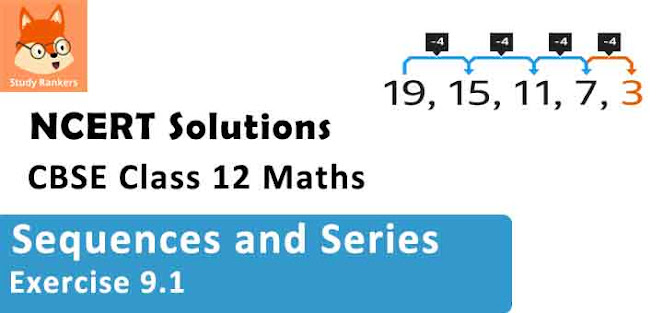### Sequences and Series Exercise 9.1 Solutions

1. Write the first five terms of the sequences whose nth term is an = n(n +2)

Solution

an = n(n+2)
Substituting n = 1, 2, 3, 4, and 5, we obtain
a1 = 1(1 +2) = 3
a2 = 2(2+2) = 8
a3 = 3(3+2) = 15
a4 = 4(4 + 2) = 24
a5 = 5(5 + 2) = 35
Therefore, the required terms are  3, 8, 15, 24, and 35.

2. Write the first five terms of the sequences whose nth term is an = n/(n+1) .

Solution

an = n/(n+1)
Substituting n = 1, 2, 3, 4, 5 we obtain
a1 = 1/(1+1) = 1/2
a2 = 2/(2+1) = 2/3
a3 = 3/(3+1) = 3/4
a4 = 4/(4+1) = 4/5
a5 = 5/(5+1) = 5/6
Therefore, the required terms are 1/2, 2/3, 3/4, 4/5, and  5/6.

3. Write the first five terms of the sequences whose nth term is an = 2n

Solution

an = 2n
Substituting n = 1, 2, 3, 4, 5 we obtain
a1 = 21 = 2
a2 = 22 = 4
a3 = 23 = 8
a4 = 24 = 16
a5 = 25 = 32
Therefore, the required terms are 2, 4, 8, 16, and 32.

4. Write the first five terms of the sequences whose nth term is an = (2n-3)/6

Solution

Substituting n = 1, 2, 3, 4, 5, we obtain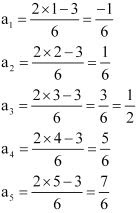Therefore, the required terms are -1/6, 1/6, 1/2, 5/6 and 7/6.

5. Write the first five terms of the sequences whose nth term is  an = (-1)n-1 5n+1

Solution

Substituting n = 1, 2, 3, 4, 5, we obtain
a1 =  (-1)1-1 51+1 = 52 = 25
a2 = (-1)2-1 52+1 = -53 = -125
a3 =  (-1)3-1 53+1 = -54 = 625
a4 = (-1)4-1 54+1 = -55 = -3125
a5 = (-1)5-1 55+1 = -56 = 15625
Therefore, the required terms are 25, -125, 625, -3125 and 15625.

6. Write the first terms of the sequences whose nth term is an = n (n2 +5)/4

Solution

Substituting n = 1, 2, 3, 4, 5 we obtain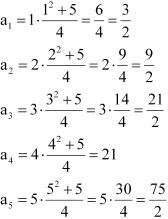Therefore, the required terms are 3/2, 9/2, 21/2, 21, and 75/2.

7. Find the 17th term in the following sequence whose nth term is an = 4n - 3: a17, a24

Solution

Substituting n = 17 , we obtain
a17 = 4(17) - 3 = 68 - 3 = 65
Substituting n = 24, we obtain
a24 = 4(24) - 3 = 96 - 3 = 93

8. Find the indicated terms in each of the sequences in Exercises 7 to 10 whose nth terms are :
an = n2 /2n ; a7

Solution

Here, an = n2 /2n
Substituting n = 7, we obtain
a7 = 72 /27 = 49/128

9. Find the 9th term in the following sequence whose nth term is an = (-1)n-1 n3 ; a9

Solution

Substituting n = 9, we obtain
a9 = (-1)9-1 (9)3 = (9)3 = 729

10. Find the 20th term in the following sequence whose nth term is an = n(n-2)/(n+3); a20

Solution

Substituting n = 20, we obtain
a20 = 20(20-2)/(20+3) = 20(18)/23 = 360/23

11. Write the first five terms of the following sequence and obtain the corresponding series:
a1 = 3, an = 3an+1 + 2 for all n > 1

Solution
a1 = 3, an = 3an+1 + 2 for all n > 1
⇒ a2 = 3a1 + 2 = 3(3) + 2 = 11
a3 = 3a2 + 2 = 3(11) + 2 = 35
a4 = 3a3 + 2 = 3(35) + 2 = 107
a5 = 3a4 + 2 = 3(107) + 2 = 323
Hence, the first five terms of the sequence are 3, 11, 35, 107, and 323.
The corresponding series is  3+11+35+107 + 323 + ....

12. Write the first five terms of the following sequence and obtain the corresponding series:
a1 = -1, an = (an -1)/n , n ≥ 2
Solution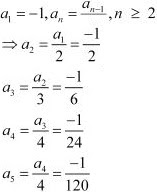Hence, the first five terms of the sequence are -1, -1/2, -1/6, -1/24 and -1/120.
The corresponding series is (-1) + (-1/2) + (-1/6) + (-1/24) + (-1/120) + ...

13. Write the first five terms of the following sequence and obtain the corresponding series:
a1 = a2 = 2, an = an-1 - 1, n > 2
Solution
a1 = a2 = 2, an = an-1 - 1, n > 2
⇒ a3 = a2 - 1 = 2 - 1 = 1
a4 = a3 - 1 = 1 - 1 = 0
a5 = a4 - 1 = 0 - 1 = -1
Hence, the first five terms of the sequence are  2, 2, 1, 0, and -1.
The corresponding series is 2 + 2 +1 + 0 + (-1) + ....

14. The Fibonacci sequence is defined by 1 = a1 = a2 and an = an – 1 + an – 2 , n > 2.
Find (an+1 )/an , for n = 1, 2, 3, 4, 5
Solution
1 = a1 = a2
an = an – 1 + an – 2 , n > 2
∴ a3 = a + a1  = 1 + 1 = 2
a4 = a + a = 2 + 1 = 3
a5 = a + a = 3 + 2 = 5
a6 = a + a = 5 + 3 = 8